# 2.7 Linear inequalities and absolute value inequalities  (Page 5/11)

 Page 5 / 11

## Verbal

When solving an inequality, explain what happened from Step 1 to Step 2:

When we divide both sides by a negative it changes the sign of both sides so the sense of the inequality sign changes.

When solving an inequality, we arrive at:

$\begin{array}{l}x+2

Explain what our solution set is.

When writing our solution in interval notation, how do we represent all the real numbers?

$\left(-\infty ,\infty \right)$

When solving an inequality, we arrive at:

$\begin{array}{l}x+2>x+3\hfill \\ \phantom{\rule{1.2em}{0ex}}2>3\hfill \end{array}$

Explain what our solution set is.

Describe how to graph $\text{\hspace{0.17em}}y=|x-3|$

We start by finding the x -intercept, or where the function = 0. Once we have that point, which is $\text{\hspace{0.17em}}\left(3,0\right),$ we graph to the right the straight line graph $\text{\hspace{0.17em}}y=x-3,$ and then when we draw it to the left we plot positive y values, taking the absolute value of them.

## Algebraic

For the following exercises, solve the inequality. Write your final answer in interval notation.

$4x-7\le 9$

$3x+2\ge 7x-1$

$\left(-\infty ,\frac{3}{4}\right]$

$-2x+3>x-5$

$4\left(x+3\right)\ge 2x-1$

$\left[\frac{-13}{2},\infty \right)$

$-\frac{1}{2}x\le \frac{-5}{4}+\frac{2}{5}x$

$-5\left(x-1\right)+3>3x-4-4x$

$\left(-\infty ,3\right)$

$-3\left(2x+1\right)>-2\left(x+4\right)$

$\frac{x+3}{8}-\frac{x+5}{5}\ge \frac{3}{10}$

$\left(-\infty ,-\frac{37}{3}\right]$

$\frac{x-1}{3}+\frac{x+2}{5}\le \frac{3}{5}$

For the following exercises, solve the inequality involving absolute value. Write your final answer in interval notation.

$|x+9|\ge -6$

All real numbers $\text{\hspace{0.17em}}\left(-\infty ,\infty \right)$

$|2x+3|<7$

$|3x-1|>11$

$\left(-\infty ,\frac{-10}{3}\right)\cup \left(4,\infty \right)$

$|2x+1|+1\le 6$

$|x-2|+4\ge 10$

$\left(-\infty ,-4\right]\cup \left[8,+\infty \right)$

$|-2x+7|\le 13$

$|x-7|<-4$

No solution

$|x-20|>-1$

$|\frac{x-3}{4}|<2$

$\left(-5,11\right)$

For the following exercises, describe all the x -values within or including a distance of the given values.

Distance of 5 units from the number 7

Distance of 3 units from the number 9

$\left[6,12\right]$

Distance of10 units from the number 4

Distance of 11 units from the number 1

$\left[-10,12\right]$

For the following exercises, solve the compound inequality. Express your answer using inequality signs, and then write your answer using interval notation.

$-4<3x+2\le 18$

$3x+1>2x-5>x-7$

$3y<5-2y<7+y$

$x+7

## Graphical

For the following exercises, graph the function. Observe the points of intersection and shade the x -axis representing the solution set to the inequality. Show your graph and write your final answer in interval notation.

$|x-1|>2$

$\left(-\infty ,-1\right)\cup \left(3,\infty \right)$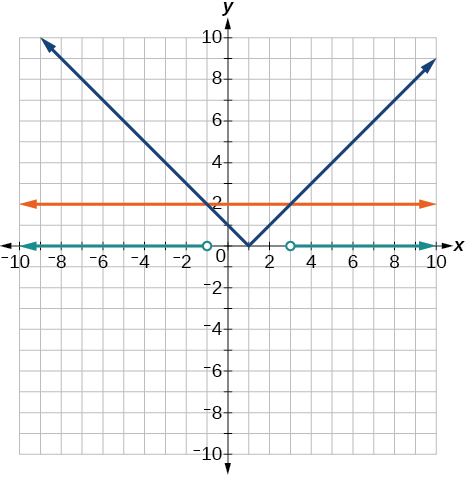$|x+3|\ge 5$

$|x+7|\le 4$

$\left[-11,-3\right]$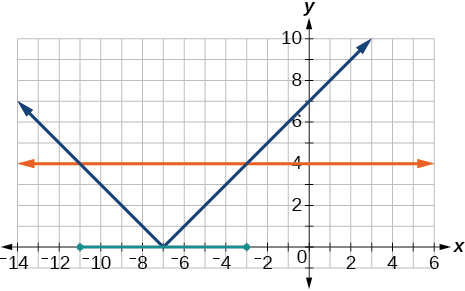$|x-2|<7$

$|x-2|<0$

It is never less than zero. No solution.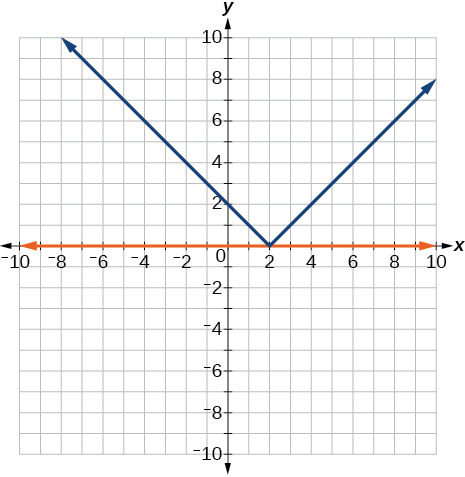For the following exercises, graph both straight lines (left-hand side being y1 and right-hand side being y2) on the same axes. Find the point of intersection and solve the inequality by observing where it is true comparing the y -values of the lines.

$x+3<3x-4$

$x-2>2x+1$

Where the blue line is above the orange line; point of intersection is $\text{\hspace{0.17em}}x=-3.$

$\left(-\infty ,-3\right)$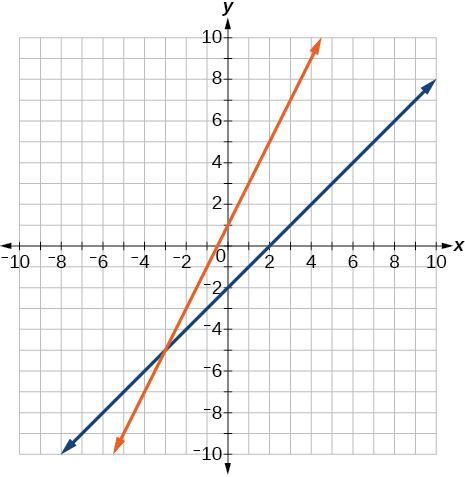$x+1>x+4$

$\frac{1}{2}x+1>\frac{1}{2}x-5$

Where the blue line is above the orange line; always. All real numbers.

$\left(-\infty ,-\infty \right)$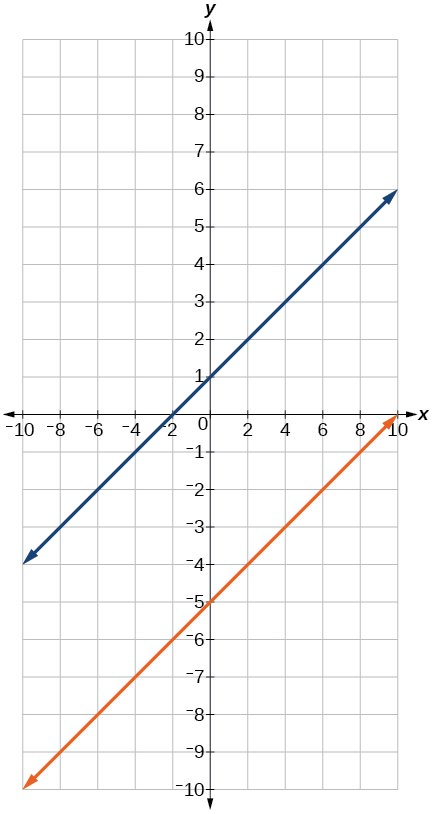$4x+1<\frac{1}{2}x+3$

## Numeric

For the following exercises, write the set in interval notation.

$\left\{x|-1

$\left(-1,3\right)$

$\left\{x|x\ge 7\right\}$

$\left\{x|x<4\right\}$

$\left(-\infty ,4\right)$

For the following exercises, write the interval in set-builder notation.

$\left(-\infty ,6\right)$

$\left\{x|x<6\right\}$

$\left(4,+\infty \right)$

$\left[-3,5\right)$

$\left\{x|-3\le x<5\right\}$

$\left[-4,1\right]\cup \left[9,\infty \right)$

For the following exercises, write the set of numbers represented on the number line in interval notation.

#### Questions & Answers

A laser rangefinder is locked on a comet approaching Earth. The distance g(x), in kilometers, of the comet after x days, for x in the interval 0 to 30 days, is given by g(x)=250,000csc(π30x). Graph g(x) on the interval [0, 35]. Evaluate g(5)  and interpret the information. What is the minimum distance between the comet and Earth? When does this occur? To which constant in the equation does this correspond? Find and discuss the meaning of any vertical asymptotes.
Kaitlyn Reply
The sequence is {1,-1,1-1.....} has
amit Reply
circular region of radious
Kainat Reply
how can we solve this problem
Joel Reply
Sin(A+B) = sinBcosA+cosBsinA
Eseka Reply
Prove it
Eseka
Please prove it
Eseka
hi
Joel
June needs 45 gallons of punch. 2 different coolers. Bigger cooler is 5 times as large as smaller cooler. How many gallons in each cooler?
Arleathia Reply
7.5 and 37.5
Nando
find the sum of 28th term of the AP 3+10+17+---------
Prince Reply
I think you should say "28 terms" instead of "28th term"
Vedant
the 28th term is 175
Nando
192
Kenneth
if sequence sn is a such that sn>0 for all n and lim sn=0than prove that lim (s1 s2............ sn) ke hole power n =n
SANDESH Reply
write down the polynomial function with root 1/3,2,-3 with solution
Gift Reply
if A and B are subspaces of V prove that (A+B)/B=A/(A-B)
Pream Reply
write down the value of each of the following in surd form a)cos(-65°) b)sin(-180°)c)tan(225°)d)tan(135°)
Oroke Reply
Prove that (sinA/1-cosA - 1-cosA/sinA) (cosA/1-sinA - 1-sinA/cosA) = 4
kiruba Reply
what is the answer to dividing negative index
Morosi Reply
In a triangle ABC prove that. (b+c)cosA+(c+a)cosB+(a+b)cisC=a+b+c.
Shivam Reply
give me the waec 2019 questions
Aaron Reply

### Read also:

#### Get the best Algebra and trigonometry course in your pocket!

Source:  OpenStax, Algebra and trigonometry. OpenStax CNX. Nov 14, 2016 Download for free at https://legacy.cnx.org/content/col11758/1.6
Google Play and the Google Play logo are trademarks of Google Inc.

Notification Switch

Would you like to follow the 'Algebra and trigonometry' conversation and receive update notifications?ByByByBy RhodesBy